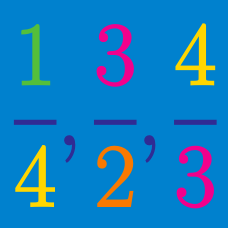Number Theory

# Rational Numbers: Level 4 Challenges

Find the smallest positive integer whose square consists of 5 identical leading digits.

As an explicit example, $149^2 = 22201$ would be the answer for 3 identical leading digits.

You have to place dots along a strip such that:

• The first two dots are in the different halves of the strip
• The first three dots are in the different thirds of the strip
• The first four dots are in the different fourths of the strip, and so on.

What is the maximum number of dots you can place on the strip?

Let $f$ be a function defined along the rational numbers such that $f(\tfrac mn)=\tfrac1n$ for all relatively prime positive integers $m$ and $n$. The product of all rational numbers $0 such that $f\left(\dfrac{x-f(x)}{1-f(x)}\right)=f(x)+\dfrac9{52}$ can be written in the form $\tfrac pq$ for positive relatively prime integers $p$ and $q$. Find $p+q$.

Let $\displaystyle \frac ab$ be the smallest of the fractions that are greater than $\displaystyle \frac {13}{15}$ where $a,b < 500$ are positive integers.

What is the value of $a+b$?

Let $a_k$ represent the repeating decimal $0.\overline{133}_k$ for $k \geq 4$. The product $a_4 a_5 \cdots a_{99}$ can be expressed as $\frac{m}{n!}$ where $m, n$ are positive integers and $n$ is as small as possible. $\frac{m}{n}$ can be expressed as $\frac{p}{q}$ where $p, q$ are coprime integers. What is $p+q$?

Note: $0.\overline{133}_k$ refers to the repeating decimal $0.133133133\ldots$ evalauted in base $k$.

×